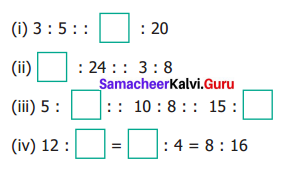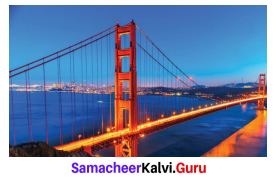# Samacheer Kalvi 6th Maths Solutions Term 1 Chapter 3 Ratio and Proportion Ex 3.3## Tamilnadu Samacheer Kalvi 6th Maths Solutions Term 1 Chapter 3 Ratio and Proportion Ex 3.3

Question 1.
Fill in the boxesSolution:
(i) 12
Hint:
5x = 3 × 20 ⇒ x = 12
(ii) 9
8x = 24 × 3 ⇒ x = 9
(iii) 4, 12
Hint:
10x = 8 × 5 = 40 ⇒ x = 4
10y = 8 × 15 = 120 ⇒ y = 12
(iv) 24, 2
Hint:
16y = 8 × 4 ⇒ y = 2
12 × 4 = 2x ⇒ x = 24

Question 2.
Say True or False.
(i) 2 : 7 :: 14 : 4
(ii) 7 Persons is to 49 Persons as 11 kg is to 88 kg.
(iii) 10 books is to 15 books as 3 books is to 15 books.
Solution:
(i) False
(ii) False
(iii) False

Question 3.
Using the numbers 3, 9, 4, 12 write two ratios that are in a proportion.
Solution:
(i) 3, 9, 4, 12
Here product of extremes = 3 × 12 = 36
Product of means = 9 × 4 = 36
3 : 9 :: 4 : 12
(ii) Also if we take 9, 3, 12, 4
Product of extremes = 9 × 4 = 36
Product of means = 3 × 12 = 36
9 : 3 :: 12 : 4

Question 4.
Find whether 12, 24, 18, 36 are in order that can be expressed as two ratios that are in proportion.
Solution:
Yes, they are in proportion
12 : 24 = 18 : 36
$$\frac{12}{24}$$ = $$\frac{1}{2}$$ Product of means = 24 × 18 = 432
$$\frac{18}{36}$$ = $$\frac{1}{2}$$ Product of extremes = 12 × 36 = 432
Hence a × d = b × cQuestion 5.
Write the mean and extreme terms in the following ratios and check whether they are in proportion.
(i) 78 litres is to 130 litres and 12 bottles are to 20 bottles
(ii) 400 gm is to 50 gm and 25 rupees is to 625 rupees.
Solution:
(i) 78 : 130 :: 12 : 20
Extreme terms are 78 and 20.
Mean terms are 130 and 12.
Product of Extremes = 78 × 20 = 1560
Product of Means = 130 × 12 = 1560
Product of Extremes = Product of means
It is in proportion.
(ii) 400 : 50 :: 25 : 625
Product of extremes = 400 × 625 = 250,000
Product of means = 50 × 25 = 1250
Here product of extremes ≠ product of means
400 : 50 and 25 : 625 are not in proportion.

Question 6.
America’s famous Golden Gate bridge is 6480 ft long with 756 ft tall towers. A model of this bridge exhibited in a fair is 60 ft long with 7 ft tall towers. Is the model in proportion to the original bridge?Solution:
The ratio of Golden Gate Bridge & Its model are = 6480 : 756
Product of extremes = 6480 × 7 = 45,360
Product of means = 756 x 60 = 45,360
Product of extremes = Product of means
The model is in proportion to the ordinal bridge.

Objective Type Questions

Question 7.
Which of the following ratios are in proportion?
a) 3 : 5, 6 : 11
(b) 2 : 3, 9 : 6
(c) 2 : 5, 10 : 25
(d) 3 : 1, 1 : 3
Solution:
(c) 2 : 5, 10 : 25

Question 8.
If the ratios formed using the numbers 2, 5, x, 20 in the same order are in proportion, then ‘x’ is
(a) 50
(b) 4
(c) 10
(d) 8
Solution:
(d) 8
5x = 2 × 20 ⇒ x = 8Question 9.
If 7 : 5 is in proportion to x : 25, then ‘x’ is
(a) 27
(b) 49
(c) 35
(d) 14
Solution:
(c) 35